Math & Beyond

# Analogy and Classification

3.9k views

 1 Introduction 2 What is meant by analogy? 3 What is Classification Analogy? 4 Description 5 Tricks or ways to solve Analogies and Classification Analogies 6 Example for Analogies and Classification from Cuemath 7 Summary 8 Conclusion 9 FAQs 10 External References

29 January 2021

An analogy is one useful method for interpreting a relationship between uncommon expressions. In this article, we will see how to solve the analogies and classification related questions and why it is important to know about it.

## What is meant by Analogy?

A cognitive process where information or meaning from a particular subject is transferred to another, this process is called Analogy. In short, the term analogy can relate to the relationship between the ‘source’ and the ‘target’ themselves.

An analogy is a comparison between two objects or systems of objects, that emphasize respects in which they are thought to be alike.

## What is Classification Analogy?

Classification analogy is one of the most common types of analogies, where there is comparing terms according to categories.

The act of grouping various objects on the basis of their common characteristics is known as classification.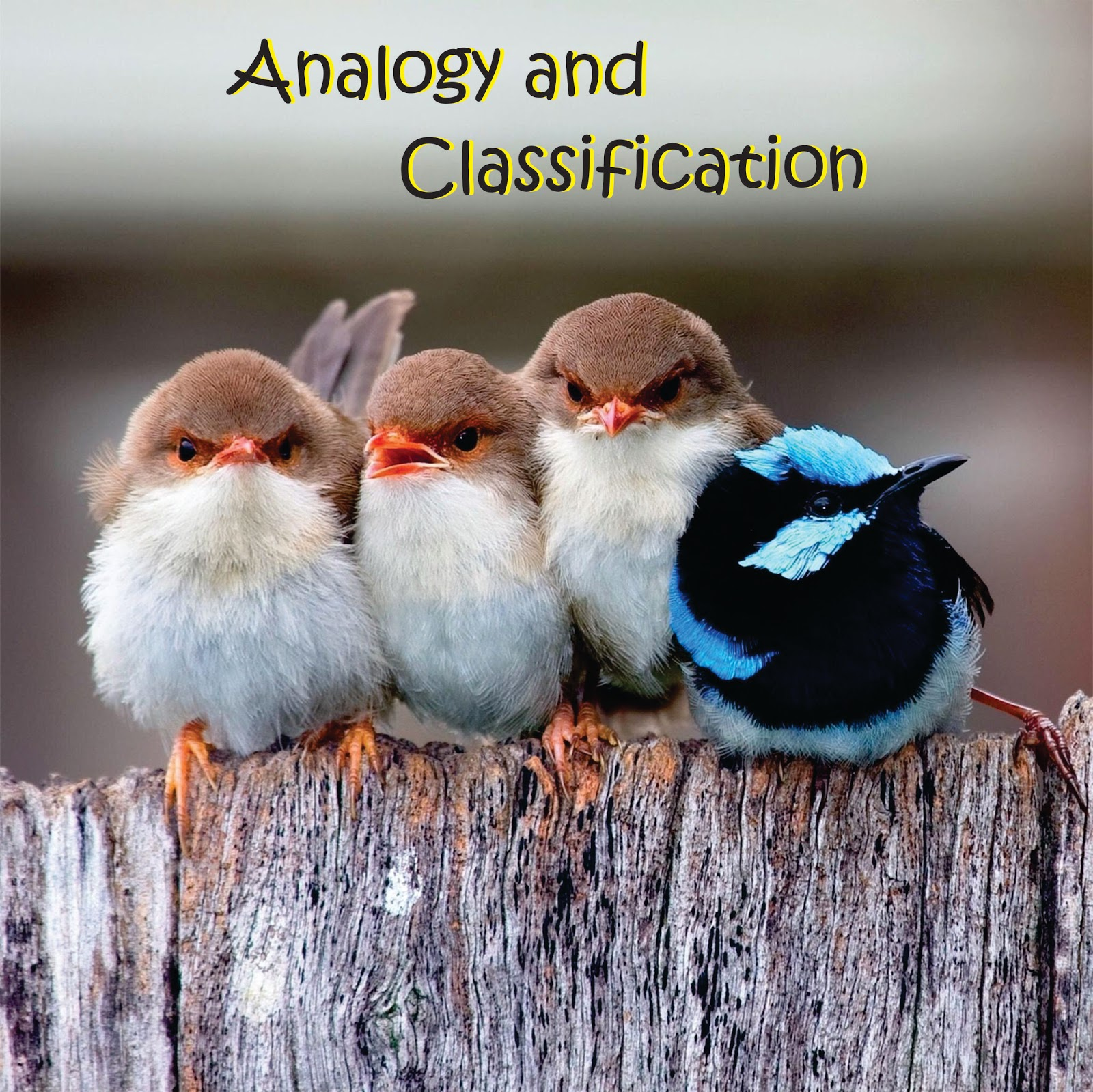In other words, a classification analogy is where there is a comparison between terms as per which group they belong to.
Let’s have a look at how one analysis, finds and solves Classification analogies. To explore more, click the downloadable PDF.

## Description

### Analogy

•  An analogy is an inference or an argument from one circumstance to another circumstance, as opposed to deduction, induction, and abduction, in which at least one of the properties, or the conclusion, is general rather than specifically in nature.
•  In analogy, we do a comparison of things, and based on those objects, we analyze whether they are alike or have many similarities.
• Analogies actually embrace the comparison or conclude on basis of reasoning itself.
• An analogy is noteworthy in fixing a problem, finding solutions, decisiveness, debate, percepts, conception, memorizing, imagination and creativity, invention, predicting and feeling, explaining and communicating.

### Classification Analogy

• So this way in classification analogies, children can demonstrate the relationship between two different pairs of objects or images, by emphasizing the classification of one of the terms as a group of another.
• Classification is done based on common properties, angle, shape, size, quantity, type, nature, colours, traits and so on.
• The rest of the elements which are common form a group and the element that has been taken out as it is different from the others in that group, as the common qualities are observed by the rest of the elements.
• Classification analogies are sometimes ranked in type, compare different levels of a ranking and also focus on relationships of insertion.
• For instance: a Dog is a type of pet animal, just as a Lion is a type of wild animal -
Dog:Pet: :Lion:Wild.
• Mostly, there are questions in which one of the terms is missing and one has to find the missing term to solve a classification analogy.
• Don’t worry as solving a classification analogy is easy if you approach it in a logical way.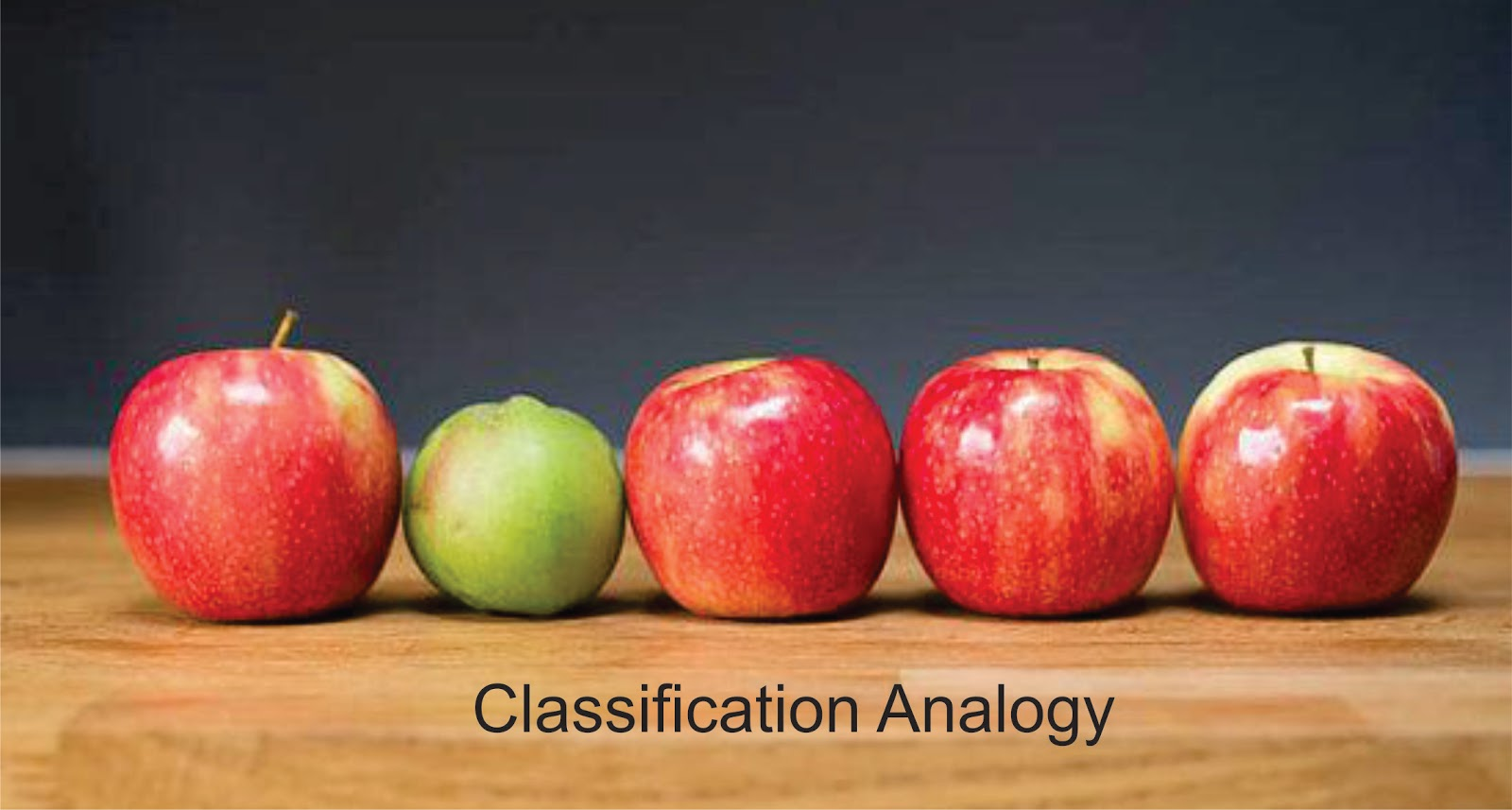Below are a few ways to solve analogy based questions. Followed by a few examples of classification analogies in which either the object or the group are missed.

## Tricks or ways to solve Analogies and Classification Analogies

• A pair of related terms/figures is provided and a similar relationship is to be established between the two other terms/figures or another pair to identify the missing term/figure.
• Try to diagnose the relationship between the first pair of terms. (Dog: Pet)
• The analogy problems can be read as sentences, even if it is actually written differently. (Dog ‘is to’ Pet just like Lion ‘is to’ Wild.)
• Change the analogies into sentences.
• Follow a systematic method especially while solving a hard problem.
• First go through answer options, before choosing a solution and not just rely on the one match that looks might be a strong match.
• Delete mentally any pairs in the answers that don’t have a similar type of relationship.
• Go through the context, keep in mind tough terms so when you are observing any analogy based questions you can recollect it and apply the logic answers.
• If the relationship of a term is unfamiliar, then try to remember the text in which you have come across the word.
• You may replace it with alternative explanations of terms.
• When solving analogies try to apply the same logic by taking another look at the relationship possibilities as mentioned above.
• If this doesn't work, discard the unlikely answers and apply logical guesses.

### While solving Classification Analogies:

• There is a set of terms/figures in which all, except one, follows similar properties.
• We are required to identify the property followed in the set and find the odd one out.
• Similar to any other analogy, follow a logical process the main aspect in being able to solve a classification analogy.
• As mentioned earlier, have a clear view of the completed part of the classification analogy.
• Try to understand the relationship between those groups.
• Follow similar steps mentioned above and check whether you can frame a sentence of that relationship.
• It is better to describe in-depth if possible the relation between two groups, but keep in mind that the other side of the analogy might have a different type of relationships.
• Now determine what is missing part that is not there in the analogy to identify the odd one.
• If something is missing, it could be a category (group), or member.
• Try to match with the completed part of the analogy, i.e. if the term is numerical - 4:20::8:40, the 4 and 8 sections will be the same (a member or a group) and the 20 and 40 sections will be the same.

Use these steps and try solving a few examples given below.

## Examples for Analogies and Classification from Cuemath

Identify the relationship and find the missing figure.

Example 1: Identify the relationship between the given pair on either side of :: and find the missing figure.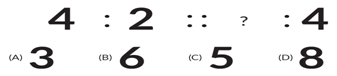(D) 8

Answer: Reason as half of digit 4 is 2, when digit 8 is divided into the half we get digit 4.

Example 2: Identify the relationship between the given pair on either side of : : and find the missing figure.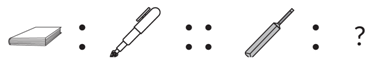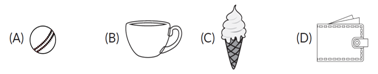(A) Ball

Answer: A pen is used to write on the book, the ball is used while playing bat.

Identify the property followed in the set and find the odd one out.

Example 1: Find the odd one out.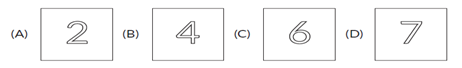(D) 7

Answer: Like all other numbers 2, 4, 6 are even numbers and divisible by 2 whereas 7 is an odd number.

Example 2: Find the odd one out.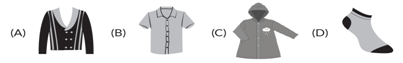(D) Socks

Answer: Reason as all other options we wear it on our upper body whereas we put socks on feet.

Exercise Questions

Question 1: Choose the fish that does not belong to the group.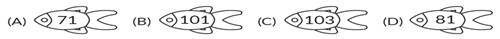(D) 81

Question 2: There is a certain relationship between the pair of figures on either side of ::. Identify the relation and find the missing figure.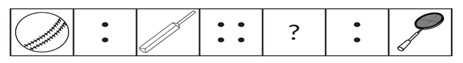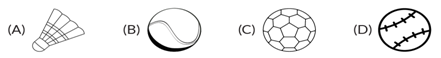(B)

Question 3: Find the odd one out.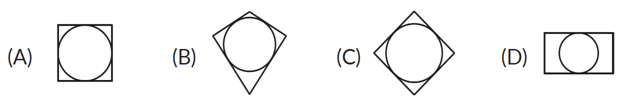(D)

Question 4: Figure (i) and (ii) are related to each other in some manner. Establish a similar relationship between figures (iii) and (iv) by selecting a suitable figure from the options.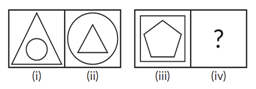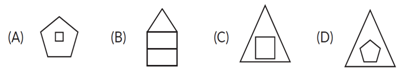(A)

Question 5: There is a certain relationship between the pair of figures on either side of :: . Identify the relationship and find the missing figure.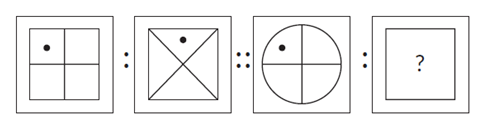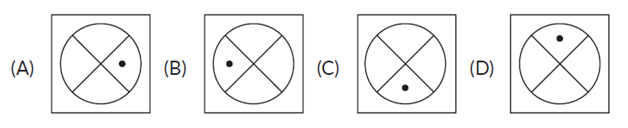(D)

Question 6: Which of the following figures is the same as the given figure?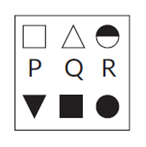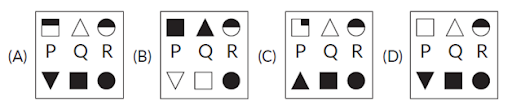(D)

Question 7: The first two figures are related. Choose an option that establishes the same rotation between the third and fourth figures.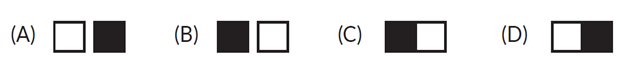(D)

Question 8: Find the odd one out.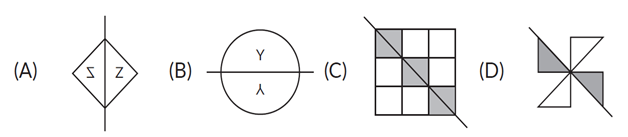(D)

## Summary

After understanding Analogy and classification well, students will be able to predict individual differences between pairs of elements. After observing the options carefully will choose the odd one out. Children will also be able to analyze figures and patterns while solving any problem. The analogy helps children develop logical thinking.

As we know practise makes one perfect, it is through practice children can be better at analogies. At Cuemath, we use these types of analogies based questions which are designed by professionals. Our main aim is to cue our students, such that they understand and solve any analogies logically and independently in the future.

## Conclusion

An analogy is a very useful trait that helps in fixing a problem, finding solutions, decisiveness, debate, percepts, conception, memorizing, imagination and creativity, invention, predicting and feeling, explaining and communicating. Thus inculcating various analogies into the children at a young age is not just beneficial but also helps them become more adaptive to the alternate conditions, and are more prone to challenges in life.

## Frequently Asked Question or FAQs

-Why successful people use analogies?

Mastering the art of the analogy can save your time, money, resources which is why successful people use them daily and understand their importance.

-What does the persuasive power of analogy, actually mean?

When it comes to creating effective understanding, analogies are hard to beat, most of which comes from the audience arriving at the intended understanding of their own. This is what is termed the persuasive power of analogy.

## External References

Related Articles
Award-winning math curriculum, FREE for a year